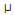# Electronics - Analog and Digital Converters - Discussion

### Discussion :: Analog and Digital Converters - General Questions (Q.No.3)

3.

A binary-weighted digital-to-analog converter has an input resistor of 100 k. If the resistor is connected to a 5 V source, the current through the resistor is:

 [A]. 50A [B]. 5 mA [C]. 500A [D]. 50 mA

Explanation:

No answer description available for this question.

 Raja said: (May 28, 2011) db/dv==100/5=20 db+30 20+30=50

 Anshu said: (Jul 25, 2011) Please explain, where you get 30 ?

 Pandhala Raju said: (Aug 29, 2011) I=V/R; =5/100K ohms =0.05m amps =50micro amps

 Pkansal2105@Yahoo.In said: (Dec 30, 2011) I=V/R =5/100000 =.05mA =50 microA

 Ilaiyaraja said: (Jan 10, 2012) I=V/R I/P resister has 100k=100000,k=1000 resister has 5v,v=5 I=5/100000 I=.00005,micro amp=10^-6 I=50microA

 Gopal Prasad said: (May 11, 2012) R=100 k V=5v I=V/R =5v/100k =0.05 mA =50 microA

 Karunakaran.R said: (Sep 28, 2014) R = 100*10^3. V = 5v. I = V/R. = 5/100*10^3. = 50*10^-6. = 50(micro)Ans.

 Dickson K Mussa said: (Dec 19, 2016) According to Ohms law. V = IR.

 Skp said: (Jun 14, 2019) What was the significance of "binary-weighted digital-to-analog converter "?